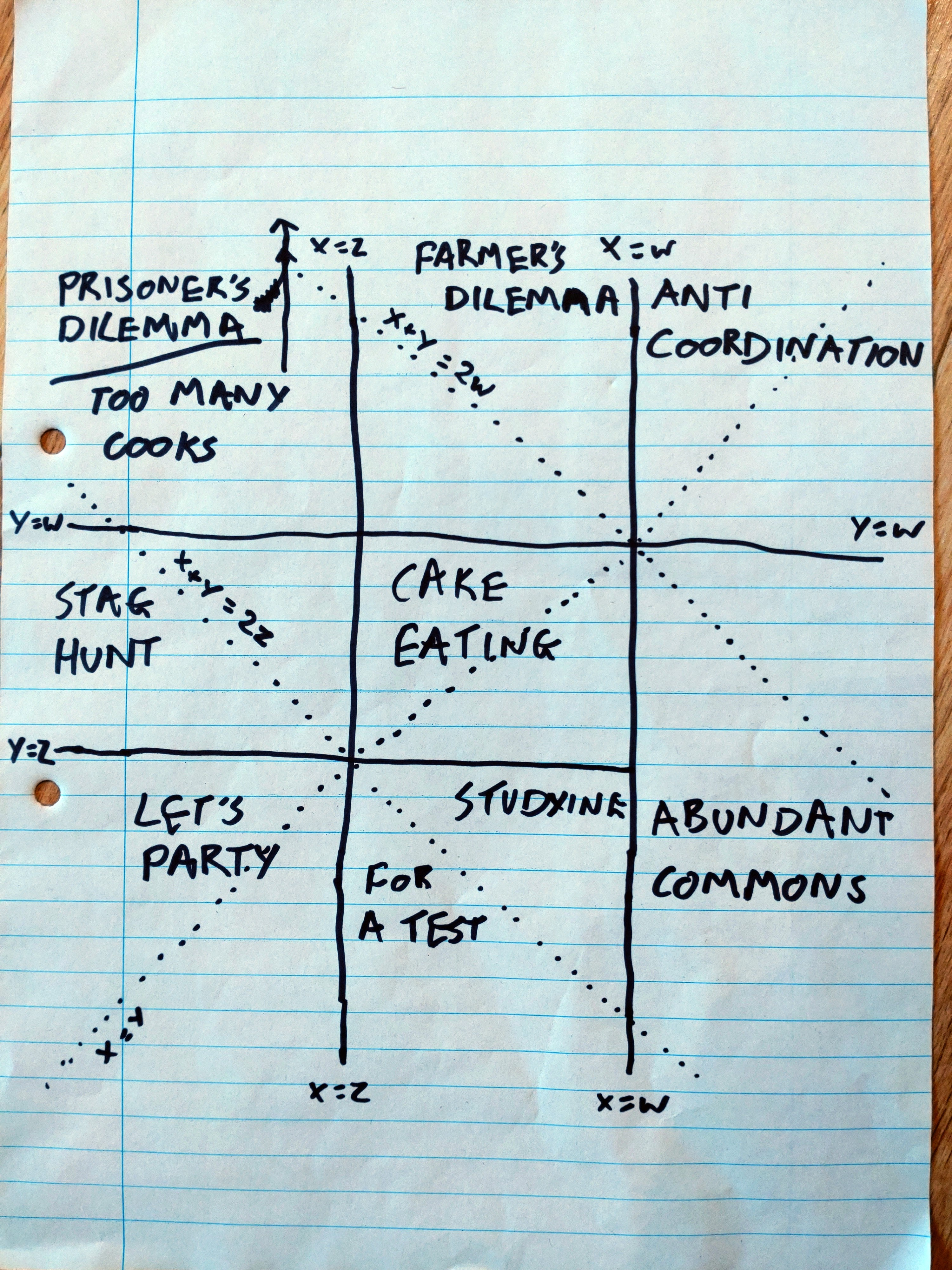# A Reasonable Approximation

### PD-alikes in two dimensions

Some time after writing Classifying games like the prisoner's dilemma, I read a paper (I forget which) which pointed out that these games can be specified with just two numbers.

Recall that they have the following payoff matrix:

Player 2
Krump Flitz
Player 1 Krump $(W, W)$ $(X, Y)$
Flitz $(Y, X)$ $(Z, Z)$

where $W > Z$.1 We can apply a positive affine transformation (that is, $n ↦ an + b$ where $a > 0$) to all of $W, X, Y, Z$ without changing the game. So let's pick the function $n ↦ {n - Z \over W - Z}$. This sends $W$ to $1$ and $Z$ to $0$, leaving us with just two parameters: $R = {X - Z \over W - Z}$ and $S = {Y - Z \over W - Z}$.

So what happens if we plot the space of these games on a graph? The lines $\{X, Y\} = \{W, Z\}$ become $\{R, S\} = \{0, 1\}$, i.e. vertical and horizontal lines. The lines $X + Y = 2W$ and $X + Y = 2Z$ become the diagonals $R + S = 2$ and $R + S = 0$; and $X = Y$ becomes the diagonal $R = S$. Drawing those lines, and relabelling in terms of $W, X, Y, Z$, it looks like this:Note that Cake Eating (my favorite game) is the only one with a finite boundary; the other boxes extend to infinity. There are also finite components in the Farmer's Dilemma (with $X + Y < 2W$), and Stag Hunt and Studying For a Test (with $X + Y > 2Z$). As drawn, Prisoner's Dilemma occupies almost all of the box it shares with Too Many Cooks; but Too Many Cooks (above the line $X + Y = 2W$) is also infinite. (I initially got those the wrong way around, so the drawing isn't very clear there.)

I don't know if we learn much from this, but here it is.

1. In the previous post I mostly ignored equalities because it was mildly convenient to do so. But the analysis here completely fails if we allow $W=Z$. So now I'm ignoring them because it's considerably more convenient to do so.

Posted on 03 April 2022

Tagged: game theory